### Testing the Parallel Lines Assumption

For an ordinal response, PROC SURVEYLOGISTIC  performs a test of the parallel lines assumption. In the displayed output, this test is labeled "Score Test for the Equal Slopes Assumption" when the LINK= option is NORMIT or CLOGLOG. When LINK=LOGIT, the test is labeled as "Score Test for the Proportional Odds Assumption" in the output. This section describes the methods used to calculate the test.

For this test, the number of response levels,, is assumed to be strictly greater than 2. Let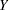be the response variable taking values. Suppose there areexplanatory variables. Consider the general cumulative model without making the parallel lines assumption: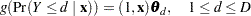whereis the link function, andis a vector of unknown parameters consisting of an intercept
andslope parameters. The parameter vector for this general cumulative model is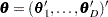Under the null hypothesis of parallelism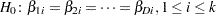, there is a single common slope parameter for each of theexplanatory variables. Letbe the common slope parameters. Letand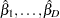be the MLEs of the intercept parameters and the common slope parameters. Then, under, the MLE of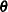isand the chi-squared score statistichas an asymptotic chi-square distribution withdegrees of freedom. This tests the parallel lines assumption by testing the equality of separate slope parameters simultaneously for all explanatory variables.